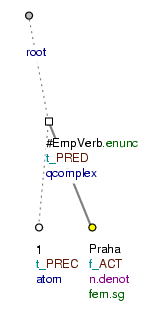### 12.7. Lists

This section introduces rules and model trees for lists of the following type:

1) Praha

2) Bratislava

3) Brno

a) povinnosti (=responsibilities / duties)

b) práva (=rights)

The following applies to the annotation of these constructions:

• a node for the empty verb is inserted into the tectogrammatical tree (`t_lemma`=`#EmpVerb`; `functor`=`PRED`).

• nodes representing numbers or letters identifying individual items in the list are assigned the functor `PREC` and are dependent on the empty verb node.

• a node representing an item in the list (or the effective root) is assigned the functor `ACT` and is dependent on the empty verb node.

Example and model tree:

1) Praha Fig. 8.241

Figure 8.241. List1) Praha (=lit. 1) Prague)